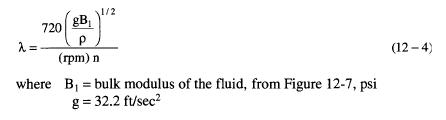# Pipe Vibrations

To minimize pipe vibrations it is necessary to design pipe runs so that the “acoustic length” of the pipe run does not create a standing wave that adds to the pressure pulsations in the system. The acoustic length is the total overall length from endpoint to endpoint including all elbows, bends, and straight pipe runs. Typical pipe runs with respect to acoustic length are considered to be:
1. Pipe length from suction tanks to the pump suction.
2. Long pipe sections between pump and pulsation dampener.
3. Pipe section between pump and manifold.

The ends of pipe runs are considered similar if both are either open or closed from an acoustic standpoint. They are dissimilar if one is open and one is closed. Examples of end classifications are:
1. If pipe size is dramatically reduced, it tends to act as a closed end.
2. Orifice plates act as closed ends.
3. Short-length flow nozzles act as closed ends.
4. Abrupt pipe diameter enlargements act as open ends.
5. Pipe tees presenting an increase in flow area (such as a tee with three equal legs) act as open ends.
6. Pipe size changes that occur smoothly over a pipe length corresponding to several pipe diameters do not act as a termination.
The acoustic wavelength is given by:p = fluid density, lb/ft3
rpm = pump speed
n = number of plungers

It is also desirable to assure that the natural frequency of all pipe spans is higher than the calculated pump pulsation frequency to minimize mechanical pipe vibrations. The pump pulsation frequency is given by:The natural frequency of pipe spans can be estimated from Figure 12-8. Normally, if the pipe support spacing is kept short, the pipe is securely tied down, the support spans are not uniform in length, and fluid pulsations have been adequately dampened, mechanical pipe vibrations will not be a problem.